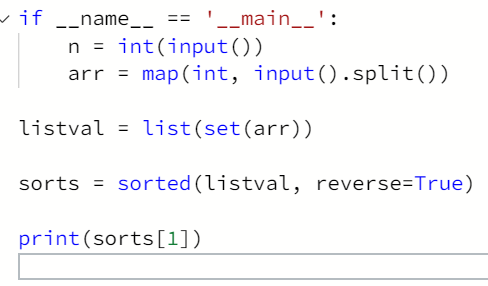# Hackerrank – Python – Data Types – Find Runner-Up Score!

Publish Date - November 11th, 2022

|

Hey there, while I grow my Python skills – I found this one specific issue to be very challenging. However, my solution is much different from the other ones online, and also works for inputs!

## The problem

Given the participants’ score sheet for your University Sports Day, you are required to find the runner-up score. You are given n  scores. Store them in a list and find the score of the runner-up.

Input Format

The first line contains n . The second line contains an array  A[] of n integers each separated by a space.

Constraints

2 <= n <= 10

-100 <= A[i] <= 100

Output Format

Print the runner-up score

``````Sample input:

5
2 3 6 6 5

Sample output:

5

Explanation

Given list is [2, 3, 6, 6, 5]. The maximum score is 6, second maximum is 5.
Hence, we print 5 as the runner-up score. ``````

## The solution

Alright, so this one definitely stumped me in the beginning – because I originally thought I could cast do the following:

• Cast the array into the list (making it easily iterable).
• Sort the list from greatest to smallest.
• Use a For loop to iterate through all of the values and then just print the second value.

After some painful unit test errors and not realizing what I was doing, I figured out that you don’t need the loop.

``````if __name__ == '__main__':
n = int(input())
arr = map(int, input().split())

listval = list(set(arr))

sorts = sorted(listval, reverse=True)

print(sorts)
``````

As you can see this is a simple three line script that passes all of the unit tests.

``listval = list(set(arr))``

This is important since you need to do two things:

1. Get rid of any duplicate values (having them doesn’t do anything for this problem).
2. Create a list so that you can easily order the list (there’s other ways to do this, but I chose to do it this way).

Once you have that value, you can run it through a sort

``````sorts = sorted(listval, reverse=True)
``````

Which for this problem should return:

``[6,5,3,2] # still a list``

Which means you need to pull a particular index out of the list, that will be consistent for other inputs:

``print(sorts)``

## Conclusion

At the end of this problem you’ve done the following steps to solve it:

1. Deduplicated the array with set(), and cast it into a list with List().
2. Ordered the list be descending value (so that the highest number is at index) with sorted().
3. Picked the second value and printed it with an index value of .

Great little question, with a pretty simple answer – but I can see where you can over think that you need a loop or list comprehension to solve this!

If you’re looking to see how I gained the skills to solve this question (without cheating), check out some of my articles below!